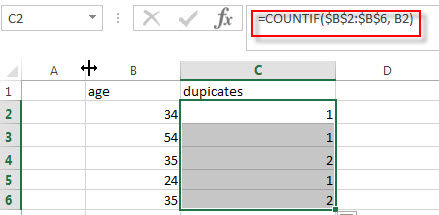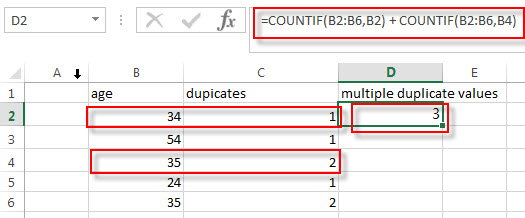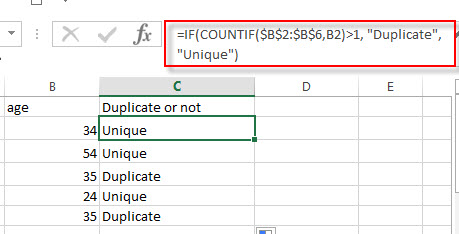# Count Duplicates

This post will guide you how to count for duplicates in Excel. You will learn how to count the instances of each duplicate value in a column.  You can use the COUNTIF function to count the duplicate values in a column in Excel.

If you have a list of data that contain duplicated values in a column, and you may want to know how many duplicates are there for each values. You can use the COUNTIF function to count the total number of cells within a selected range of cells which match a given criteria. The syntax of the COUNTIF function is as below:

`= COUNTIF (range, criteria)`
• Range -This is a required argument.  The range of cells that you want to apply the criteria to count
• Criteria – This is a required argument.  The criteria used to define which cells are counted

## Count Duplicates values in a column

Assuming that you want to count duplicates in column B for each of those values, you can create an excel formula based on the COUNTIF function as follows:

`=COUNTIF(\$B\$2:\$B\$6, B2)`You need to provide an absolute cell reference for the range of cells that you need to count all the duplicates in. so the range value can be set as: \$B\$2:\$B\$6.

If you need to count the total number of duplicates for two or more values in a column, for example, you maybe need to count how many times two sets of values are duplicated within a cell range. You just need to add one more COUNTIF formula, such as:

`=COUNTIF(B2:B6,B2) + COUNTIF(B2:B6,B4)`## Duplicates value checking

If you want to check if the value in a cell is duplicated. If duplicated, then returns duplicate message, otherwise, returns unique message. You can create the below formula based on the IF function and COUNTIF function to check duplicates in a range of cells B2:B6.

`=IF(COUNTIF(\$B\$2:\$B\$6,B2)>1, "Duplicate", "Unique")`### Related Functions

• Excel COUNTIF function
The Excel COUNTIF function will count the number of cells in a range that meet a given criteria. This function can be used to count the different kinds of cells with number, date, text values, blank, non-blanks, or containing specific characters.etc.= COUNTIF (range, criteria)…
• Excel IF function
The Excel IF function perform a logical test to return one value if the condition is TRUE and return another value if the condition is FALSE. The IF function is a build-in function in Microsoft Excel and it is categorized as a Logical Function.The syntax of the IF function is as below:= IF (condition, [true_value], [false_value])….

### Related Posts

• Count the number of words in a cell
If you want to count the number of words in a single cell, you can create an excel formula based on the IF function, the LEN function, the TRIM function and the SUBSTITUTE function. ..
• Get the First Match in Two Excel Ranges
If you want to find the first match between two excel ranges, you can use a combination of the INDEX function, the MATCH function and COUNTIF function to create a new formula….
•  Extract a List of Unique Values from a Column Range
If you want to extract a list of unique items from a column or range, you can use a combination of the IFERROR function, the INDEX function, the MATCH function and the COUNTIF function to create an array formula….